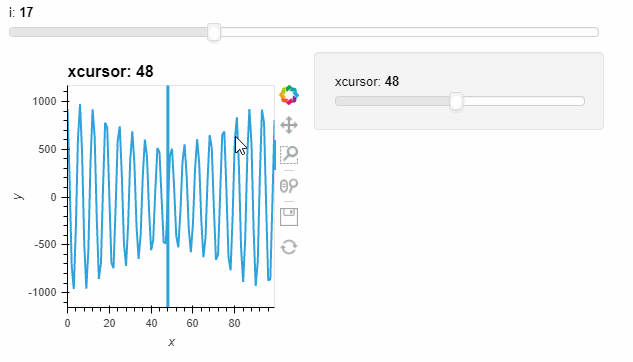# Framewise per elements

Hello,

I’d like to know if there is a way to apply the framewise option only to one element in an overlay of DynamicMaps

``````import numpy as np; import panel as pn; import holoviews as hv; hv.extension('bokeh'); pn.extension();
r = np.arange(100*40).reshape([40,100])
sine = np.exp(-(r-2000)**2/2000)*np.sin(r)
curves = [[(il+ix)**3*sine for ix in range(50)] for il in range(50)]
s = pn.widgets.IntSlider(value=0, start=0, end=49, name='i')
@pn.depends(i=s)
def plot(i):
mean = np.mean(curves[i][i],axis=0)
return hv.Curve(mean)

dmap = hv.DynamicMap(plot).opts(axiswise=False, framewise=True)
dmap_cursor = hv.DynamicMap(lambda xcursor: hv.VLine(xcursor), kdims='xcursor').redim.range(xcursor=(0,99))
pn.Column(s, dmap*dmap_cursor)
``````

For example I would like to auto scale axis when the slider `s` is moved but not when the xcursor is changed